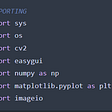# Let’s do it…

## Code for how to detect shapes using cv2…

`import cv2import numpy as npimg = cv2.imread('someshapes.jpg')cv2.imshow('1. original image', img)cv2.waitKey(0)gray_img = cv2.cvtColor(img, cv2.COLOR_BGR2GRAY)cv2.imshow('2. gray image', gray_img)cv2.waitKey(0)edges = cv2.Canny(gray_img, 50, 200)cv2.imshow('3. edges', edges)cv2.waitKey(0)contours, hierarchy = cv2.findContours(edges.copy(), cv2.RETR_EXTERNAL, cv2.CHAIN_APPROX_NONE)for cnt in contours:    approx = cv2.approxPolyDP(cnt, 0.03*cv2.arcLength(cnt, True), True)    print(len(approx))    # detect shapes using cv2    if len(approx) == 3:        shape = 'Triangle'        M = cv2.moments(approx)        cx = int(M['m10']/M['m00'])        cy = int(M['m01']/M['m00'])    elif len(approx) == 4:        x, y, w, h = cv2.boundingRect(cnt)        if abs(w-h) < 5:            shape = 'Square'            M = cv2.moments(approx)            cx = int(M['m10'] / M['m00'])            cy = int(M['m01'] / M['m00'])        else:            shape = 'Rectangle'            M = cv2.moments(approx)            cx = int(M['m10'] / M['m00'])            cy = int(M['m01'] / M['m00'])    elif len(approx) == 10:        shape = 'Star'        M = cv2.moments(approx)        cx = int(M['m10'] / M['m00'])        cy = int(M['m01'] / M['m00'])    elif len(approx) == 8:        shape = 'Circle'        M = cv2.moments(approx)        cx = int(M['m10'] / M['m00'])        cy = int(M['m01'] / M['m00'])    cv2.putText(img, shape, (cx-30, cy),cv2.FONT_HERSHEY_SIMPLEX, 0.7, (0, 255, 0), 1)    cv2.drawContours(img, cnt, -1, (0, 255, 0), 2)    cv2.imshow('cnt', img)cv2.destroyAllWindows()`
• Line 1–2 — Importing required libraries.
• Line 4–6 — Show the input image, till someone hits any key.
• Line 8–10 — Convert the image to grayscale and show the image.
• Line 12 -14 — Detect edges from the gray image, and show them.
• Line 16 — Detect contours from the image with edges.
• Line 18 — Let’s traverse in contours.
• Line 20 — Calculate the number of lines in contours using cv2.approxPolyDP() and let’s detect shapes using cv2.
• Line 23–27 — If no. of lines equals 3, then it’s a triangle.
• Line 29–40 — If the no. of lines equals 4 then it’s either a rectangle or a square.
• Line 31–35 — If the difference between height and width is less than 5 pixels, then there’s a very negligible difference in width and height, it’s a square.
• Line 36–40 — Else it’s a rectangle.
• Line 42–46 — If no. of lines equal 10, it’s a star.
• Line 48–52 — If no. of lines equal 8, it’s a circle.
• Line 54 — Put the text in the center of every detected contour. We calculated the center coordinates of every contour using moments.
• Line 56 — Draw the contours and show the image.
• Line 58 — Show the output image.
• Line 59 — Destroy all the open windows.

--

--

--

## More from Abhishek Sharma

Data Scientist || Blogger || machinelearningprojects.net

Love podcasts or audiobooks? Learn on the go with our new app.

## Feature Deployments in Kubernetes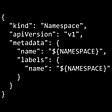## How to Stand Out as a Software Engineer in 2021 — Insights & Advice## How I Got the Summer Internship Offer from Facebook## Coding like a human## Azure Blob Storage Upload File.Net Core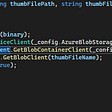## What I learned about databases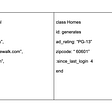## Abhishek Sharma

Data Scientist || Blogger || machinelearningprojects.net

## Outro To OpenCV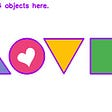## How to Split and Merge Color Channels in cv2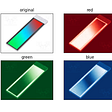## Fake Account Detection using TensorFlow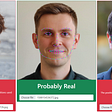## Cartoonize an Image using OpenCV